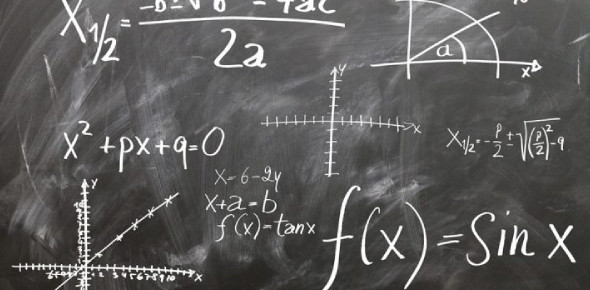# Algebra Quiz: Test Your Skills!

10 Questions | Total Attempts: 78SettingsCreate your own QuizDo you think you know algebra? Would you be willing to try this quiz? Algebra is the study of mathematical symbols and the rules for shaping these symbols. It represents a connection between itself and all types of mathematics. The more essential parts of algebra are called elementary algebra. The more advanced algebra is called abstract algebra or modern algebra. If you want to put your algebra skills to the test, stop right here and take this quiz.

• 1.
Simplify the following (do not leave spaces in your answers) a) 6a + 7b + 3a + 9b = [Blank]  b) 10a + 9b + a + 5b = [Blank] c) a + 8b + 7a + 2b = [Blank]  d) a + 2b + 2 + 4a + 6b + 1 = [Blank] e) 10a + 9b + 2 + 4a + 7b + 5 = [Blank] f) 3a - 9b + 3 - 9a + 8b + 4 = [Blank] g) -10a + 10b + 3 + 3a + 5b + 1 = [Blank] h) 7a - 8b + 10 + 7a - 4b + 8 = [Blank] i) 8a - 2b + 2 + 7a - 10b + 4 = [Blank] j) 10a + 5b + 7 - 2a - 2b + 2 = [Blank]
• 2.
Multiply out: a) 4(7z - 10) = [Blank]  b) 10(-9e - 5) = [Blank] c) 6(4f - 7) = [Blank] d) 9(-4g + 10) = [Blank] e) 2(7x - 4) = [Blank]
• 3.
Multiply out and simplify the following: a) 2(9b + 4) + 4(8b + 6) = [Blank]  b) 5(6y + 7) + 4(y + 3) = [Blank] c) 5(8k + 9) + 4(5k + 9) = [Blank] d) 2(3c + 1) + 5(6c + 9) = [Blank] e) 3(3v + 8) + 5(4v + 9) = [Blank]
• 4.
Multiply out and simplify the following: a) 5(4m + 6) - 10(9m + 2) = [Blank] b) 3(6h + 3) - 10(5h + 7) = [Blank] c) 10(7e + 8) - 8(3e + 2) = [Blank] d) 4(2q + 8) - 9(7q + 8) = [Blank] e) 8(s + 6) - (4s + 5) = [Blank]
• 5.
Work out the following: a) 10w + 9z when w = 9 and z = -6. [Blank] b) 6n - 8j when n = -2 and j = -2. [Blank] c) 5a + r when a = -1 and r = 10. [Blank] d) f - 8g when f = 10 and g = -6. [Blank] e) 4k + 7m when k = -8 and m = -3. [Blank]
• 6.
Solve the following: a) 9e - 9 = 3e - 15    e=[Blank]  b) 3v + 28 = 8v - 2    v = [Blank] c) 9b + 6 = 8b - 1     b = [Blank] d) 6p + 9 = 5p + 13   p = [Blank] e) 2n - 10 = 3n - 5     n = [Blank]
• 7.
Solve the following equation 5(2a+3) = 25 a = [Blank]
• 8.
Solve the equation a=[Blank]
• 9.
Solve the equation a=[Blank]
• 10.
Solve the equation 2(3a - 5) - 5(2a + 3) = 15 a=[Blank]
Related TopicsBack to top
×

Wait!
Here's an interesting quiz for you.